Quantity: 0

Total: 0,00

0

# Grouping of solids

### Grouping of solids

This animation demonstrates various groups of solids through examples.

Mathematics

Keywords

grouping of solids, cylindrical objects, Rectangular prisms, prism, cuboid, cube, cylindrical, set, rectangular, solids, mathematics

Related items

### Related items#### Grouping of cuboids

This animation demonstrates various types of cuboids through everyday objects.#### Building shapes (one colour)

Build 3D shapes from unit cubes with the help of several views.#### Conic sections

The conic section is a plane curve that is created when a right circular cone is intersected by a plane.#### Conic solids

This animation demonstrates various types of cones and pyramids.#### Cube

This animation demonstrates the components (vertices, edges, diagonals and faces) of the cube, one of the Platonic solids.#### Cuboid

A cuboid is a polyhedron with six rectangular faces.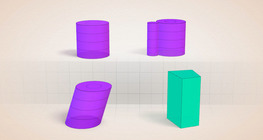#### Cylindrical solids

This animation demonstrates various types of cylindrical solids as well as their lateral surfaces.#### Grouping of solids 1

This animation demonstrates various groups of solids through examples.#### Grouping of solids 2

This animation demonstrates various groups of solids through examples.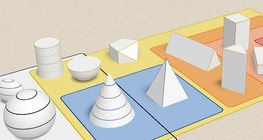#### Grouping of solids 3

This animation demonstrates various groups of solids through examples.#### Grouping of solids 4

This animation demonstrates various groups of solids through examples.#### Perimeter, area, surface area and volume

This animation presents the formulas to calculate the perimeter and area of shapes as well as the surface area and volume of solids.#### Platonic solids

This animation demonstrates the five regular three-dimensional (or Platonic) solids, the best known of which is the cube.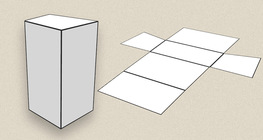#### Prisms

This animation demonstrates several types of prisms, from general to regular.#### Ratio of volumes of similar solids

This 3D scene explains the correlation between the ratio of similarity and the ratio of volume of geometric solids.#### Solids of revolution

Rotating a geometric shape around a line within its geometric plane as an axis results in a solid of revolution.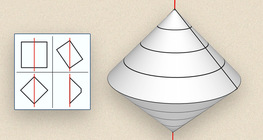#### Solids of revolution (exercise)

An exercise about the generation of solids of revolution.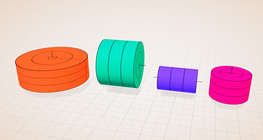#### Solids of revolution (rectangle)

Rotating a rectangle around its axes of symmetry or around its sides results in solids of revolution.#### Sphere

A sphere is the set of points which are all within the same distance from a given point in space.